# SAT Math Multiple Choice Question 990: Answer and Explanation

### Test Information

Question: 990

10. Right triangle ABC has side lengths 7, 24, and 25. If ∠B is the second-largest interior angle of the triangle, what is the cosine of ∠B?

• A.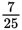• B.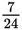• C.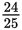• D.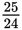Explanation:

A

Difficulty: Easy

Category: Additional Topics in Math / Trigonometry

Strategic Advice: The second-largest angle of a triangle must be opposite the second-longest side. Knowing this allows you to draw a sketch and use SOH CAH TOA to find the cosine of the indicated angle.

Getting to the Answer: Draw a quick sketch of a right triangle with one leg that is considerably longer than the other. (The hypotenuse of a right triangle is always the longest side.) The sketch should help you see that ∠B is across from the side labeled 24: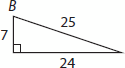By SOH CAH TOA, cos ∠B =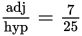, which is (A).